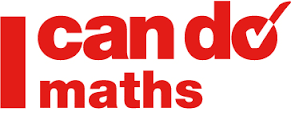# Maths Paper for All Competitive Tests – Quantitative Aptitude Mock Test

Math Paper for All Competitive Exams ( SSC, HSSC, Railways, IBPS ( Banking Sector )

Math ( Quantitative Aptitude ) is an integral part of all the major Competitive Exams in the country. You can’t escape it. So it is in the fitness of the thing that you must be ready for it. Maths is a skilled subject and most of it relies upon consistent practice. We are making earnest endeavor to publish Maths Paper that shall be very helpful for all the Competitive Exams such as IBPS PO/ Clerk, SSC CGL/ CHSL, Railways, LIC, GIC etc. So Check your Practice of Mathematics ( Quantitative Aptitude ) from below.Math Paper for all Exams –

Q.1 What is the least no by which 825 must be multiplied in order to produce a multiple of 715 ?

a.13 b. 17 c. 11 d. 16

Q. 2. Find the greatest no of 4 digits and the least no of 5 digits that have 144 as their HCF ?

a.10,000 b. 10,080 c. 11,080 d. 9,998

Q. 3. In how many words can be the letters of the word INTERMEDIATE be arranged ?

a. 19999 b. 199589  c. 19958400  d.19945800

Q. 4. A and B enters into a partnership with capitals as 5:6, and at the end of 8 months, A withdraws. If they receive profits in the ratio of 5:9, find how long B’s Capital was used ?

a. 10 Months b. 11 Months  c. 9 Months d. 12 Months

## Quantitative Aptitude Test for SSC/ IBPS Exams

Q. 5. 40 liters of a mixture of milk and water contain 10% of water. How much water must be added to make the water 20% in the new mixture ?

a. 4 liters b. 6 liters c. 5 liters d.7 liters

Q. 6. The age of a man is 4 times that of his son. 5 years ago, the man was 9 times as old as his son was at that time. What is the present age of the man ?

a. 32 yrs b. 34 yrs c. 36 yrs d.30 yrs

Q. 7. Joseph purchased a horse at 9/10 of its selling price and sold it at 8% more than its selling price. Find his gain per cent ?

a. 10% b. 15 % c. 20% d.24%

Q. 8. There would be 10% loss if rice is sold at Rs. 5.40 per kg. At what price per kg should it be sold to earn a profit of 20% ?

a.6.2 per kg b. 7.2 per kg  c. 10.8 per kg d.9.2 per kg

Q. 9. A horse worth Rs 9000 is sold by A to B at 10% loss. B sells the horse back to A at 10% gain. Who gains ?

a. a – 10% b. b – 10%  c. a – 5% d. b – 5%

### Maths Practice Papers

Q. 10 A man sells an article at 5% profit. If he had bought it at 5% less and sold it for Re. 1 less, he would have gained 10%. Find the cost price ?

a. Rs 200 b. R. 100 c. Rs. 150 d. Rs 250

Which of the following does not fit in the series ? ( from Questions 11 to 15 )

Q. 11. 7, 10, 12, 14, 17, 19, 22, 22

a. 10 b. 17 c. 19 d. 22

Q. 12. 2185,727. 241, 79, 30, 7, 1

a. 2185 b. 241 c. 30 d. 1

Q. 13. 2, 1, 10, 19, 14, 7, 16

a.2 b. 1 c. 10 d. 19

Q. 14 1, 1, 1, 4, 2, 1, 9, 5, 1, 16

a.14 b. 1 c. 2  d. 5

Q. 15  -1, 5, 20, 59, 119, 209, 335

a. 20 b. 119 c. 209  d. 335

Ans – 1. a 2. b 3.c 4. d 5. c 6. a. 7. c 8.b 9. b 10. a 11. c 12. c 13. d 14 d 15. a

Practice Maths Set – 1 for all Exams

Maths Paper Set – 2

Practice Set on LCM and HCF

Time and Work Chapter

Practice paper on Profit and Loss

Important Papers for Upcoming Exams –

Practice English Papers from here.

Reasoning Mock Paper

Test your General Knowledge from this Paper